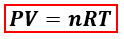# Problem: A 2.50-L container is filled with 175 g argon.a. If the pressure is 10.0 atm, what is the temperature?

###### FREE Expert Solution

We are asked to find the temperature at the given conditions. Since mass, volume and pressure are given, we will use the ideal gas equation to calculate for temperature.P = pressure, atm
V = volume, L
n = moles, mol
R = gas constant = 0.08206 (L·atm)/(mol·K)
T = temperature, K

In the ideal gas equation, moles are needed but the mass of Argon (Ar) is given instead. We’ll calculate the moles of Ar using its mass and molar mass.

90% (332 ratings)###### Problem Details

A 2.50-L container is filled with 175 g argon.
a. If the pressure is 10.0 atm, what is the temperature?